# Exercise: Modeling and simulation of an exothermic reaction

(Contributed by Anand Asthagiri, 3 Oct 04)

Consider a chemical reactor in which species A undergoes a first-order, exothermic conversion to species B. To remove the heat of reaction, a jacket surrounds the reactor where a coolant is maintained at 100 deg F. Suppose that such a reactor is performing at steady-state conditions provided in the table below: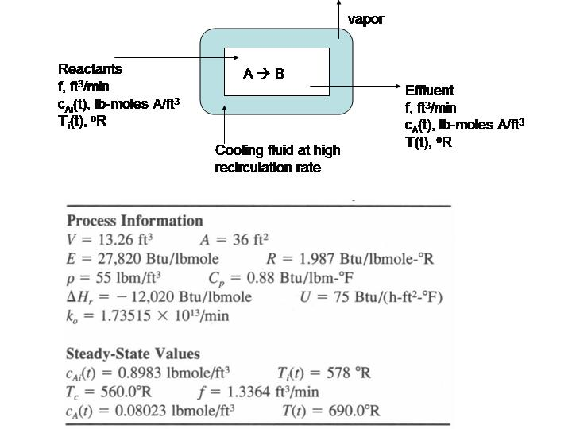Inevitably, under normal process conditions, the reactor will experience disturbances in the inlet temperature () and concentration of species A () in the input stream. Thus, we would like to know what impact these fluctuations in inlet conditions might have on the concentration of species A (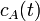) and the temperature () of the effluent stream.

Suggestions: Assume that the reactor contents are well-mixed and that the heat capacity (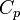) and density () of reactants and products are equal.

1. Develop a set of equations that could be used to predict temporal changes in effluent temperature and species A concentration (and, respectively).

2. Since we are interested in deviations in process variables, it is useful to reformulate the above equations in terms of deviation variables. A deviation variable () for a process variable (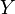) is defined aswhereis the steady-state value. Reformulate equations in terms of such deviation variables, and solve forand. </li>

3. Plotandversus time for the following cases: (a)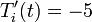deg R and (b)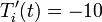deg R. Explain the observed behavior of the reactor. Does it always return to the same steady-state value? Is the dynamic response ``smooth or oscillatory? </li>